# LOGIC FRACTALS

A number of interesting patterns can be created using the bitwise logic operators (AND (&) OR (|) and XOR (^)). I call these patterns "Logic Fractals", since they exhibit self similarity at different scales.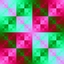Plaid Bits The basic pattern is the result of the underlying generator (X XOR Y), which is also used to generate a number of the images in the "logic fractals" group.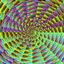Polar Dartboard This pattern creates a logic fractal based on the polar coordinates D and A. D is the distance from the center, and A is the angle, from 0 to 2*PI. In this case the angle is scaled to create a sweep from 0 to 255.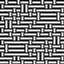XOR Weave The COND() function is used to alternate between two different gradient patterns. The condition used for screening is (X XOR Y) MOD 3.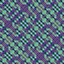XOR 12-24-48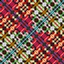XOR Movie This logic fractal animates over time, and makes an interesting movie.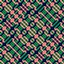XOR Zoom A zoom sequence intended to demonstrate the self similar qualities of a logic fractal.